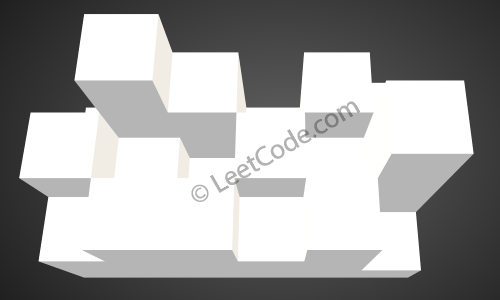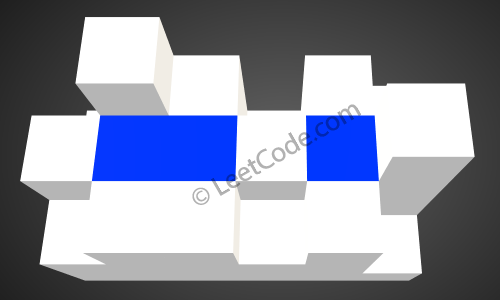# LeetCode_407: Trapping Rain Water II

一道很有意思的题目，题目是计算容器雨水积累量，实际上是利用广度优先搜索来不断收缩边界，计算雨水的积累。由于博主之前图一类的题目接触的比较少，这道题目看了一眼答案。这篇博客除了介绍这道题目本身，还将介绍stl中的优先队列priority queue，优先队列是广度优先搜索（BFS）必要的辅助。作为编程辅助，后面还顺便介绍了C++11的花括弧初始化。

### STL优先队列

#### priority_queue的使用

1. 需要在头文件中#include<queue>
2. 默认采用大优先级队列（大顶堆）
3. 优先队列中只使用了小于号（<），如果需要使用小优先级队列（小顶堆），只需要重载小于号就可以了
4. 还有一种使用小优先级队列的方法，就是在构造时指定比较函数，这优点类似于sort中的自定义比较大小函数，后面的代码中会使用这种方法
//定义结构，使用运算符重载,自定义优先级1
struct cmp1{
bool operator ()(int &a,int &b){
return a>b;//最小值优先
}
};
struct cmp2{
bool operator ()(int &a,int &b){
return a<b;//最大值优先
}
};
//定义结构，使用运算符重载,自定义优先级2
struct number1{
int x;
bool operator < (const number1 &a) const {
return x>a.x;//最小值优先
}
};
struct number2{
int x;
bool operator < (const number2 &a) const {
return x<a.x;//最大值优先
}
};

int main()
{   priority_queue<int>que;//采用默认优先级构造队列, 默认使用vector做容器

priority_queue<int,vector<int>,cmp1>que1;//最小值优先
priority_queue<int,vector<int>,cmp2>que2;//最大值优先

priority_queue<int,vector<int>,greater<int> >que3;//注意“>>”会被认为错误，  greater为函数从大到小排序
//这是右移运算符，所以这里用空格号隔开
priority_queue<int,vector<int>,less<int> >que4;////最大值优先 less则相反
...

#### priority_queue的方法

priority_queue调用了STL中的make_heap(), pop_heap(), push_heap()等操作实现了一个优先队列，如上面贴的代码，在构造时，必须声明类型，可以选择声明容器（一般使用默认的vector，也可以使用dqueue，如果使用list编译不报错，但是无法使用），同时还可以选择比较函数，在选择比较函数时，默认使用less（大值优先）。

• empty()，是否空，true对应空
• push(a)，将a元素送入队列
• pop()，将最大元素删除
• top()，返回最大元素（没有删除）
• size()，返回元素个数
• front()，back()是由queue提供的方法

#### C++11特性：统一花括弧初始化

struct A
{
int x;
int y;
};

A a{123,456};  //a.x=123; a.y=456

//下面三行来自C++03：
int a1=1;
int a2=int(1);
int a3(1);
//下面是C++11新增的：
int a4={1};
int a5{1};

A a2=A(1,2);
A a3(1,2);
A a4={1,2};
A a5{1,2};

C++11还增加了新特征

A func()
{
//C++03风格：
return A(123,456);
//C++11风格：
return {123,456};
}

long double ld = 3.1415926536；
int a {ld}, b = {ld}    //编译器报错，存在丢失信息的风险
int c (ld), d = ld ;    //正确

vector<vector<int>> dir{ { 0, -1 }, { -1, 0 }, { 0, 1 }, {1, 0} };
vector<vector<int>> heights = {
{12, 13, 0, 12},
{13, 4, 13, 12},
{13, 8, 10, 12},
{12, 13, 12, 12},
{13, 13, 13, 13}
};

### 题目

Given an m x n matrix of positive integers representing the height of each unit cell in a 2D elevation map, compute the volume of water it is able to trap after raining.

Note:
Both m and n are less than 110. The height of each unit cell is greater than 0 and is less than 20,000.

Example:

Given the following 3×6 height map: [ [1,4,3,1,3,2], [3,2,1,3,2,4], [2,3,3,2,3,1] ] Return 4.### 分析

1. 将最外围边界加入最小优先队列。
2. 从最低点开始进行BFS，最低点周围填充高度肯定等于最低点。
3. 搜索过程中不断更新边界（当前搜索出发点出队，搜索点周围点入队），并更新边界内可储水高度。
4. 每个位置的水量等于可储水高度减去底部高度。每个格子进行累加，即为总储水高度。

### 解答

class Solution{
public:
int trapRainWater(vector<vector<int>>& heightMap){
int val = 0;
const int cols = heightMap.size();
if (cols < 3){
return 0;
}
const int rows = heightMap.size();
if (rows < 3){
return 0;
}
priority_queue<pair<int, int>, vector<pair<int, int>>, greater<pair<int, int>>> q;
for (int i = 0; i < cols; ++i){
q.push({heightMap[i], i * rows});
q.push({heightMap[i][rows-1], i * rows + rows-1});
}
for (int i = 1; i < rows - 1; ++i){
q.push({heightMap[i], i});
q.push({heightMap[cols-1][i], (cols-1)*rows + i});
}

// bfs
vector<vector<int>> dir{ {1, 0}, {-1, 0}, {0, 1}, {0, -1} };
vector<vector<bool>> visited(cols, vector<bool>(rows, false));
int mx = INT_MIN;
while (!q.empty()){
const auto t = q.top(); q.pop();
mx = max(mx, t.first);

const int c = t.second / rows; //xx
const int r = t.second % rows; //yy
for (int i = 0; i < 4/*dir.size()*/; ++i){
const int x = c + dir[i];
const int y = r + dir[i];
if (x < 1 || x >= cols-1 || y < 1 || y >= rows-1 || visited[x][y]){
continue;
}
visited[x][y] = true;
if (heightMap[x][y] < mx){
val += mx - heightMap[x][y];
}
q.push({heightMap[x][y], x * rows + y});
}
}
return val;
}
};